Refer to our Texas Go Math Grade 3 Answer Key Pdf to score good marks in the exams. Test yourself by practicing the problems from Texas Go Math Grade 3 Module 7 Assessment Answer Key.

Choose the best term from the box to complete the sentence.

Question 1.
A ___ of 4 is any product that has 4 as one of its factors. (p. 217)
Explanation:
A number that may be divided by another a certain number of times without a remainder.

Question 2.
This is an example of the ___ Property.
3 × 8 = (3 × 6) + (3 × 2)
This property states that multiplying a sum by a number is the same as multiplying each addend by the number and then adding the products. (p.229)
Distributive property
Explanation:
The distributive property of multiplication can be used when you multiply a number by a sum.
For example,
suppose you want to multiply 3 by the sum of 10 + 2.

Concepts and Skills

Write one way to break apart the array. Then find the product. TEKS 3.4.E, 3.4.K

Question 3.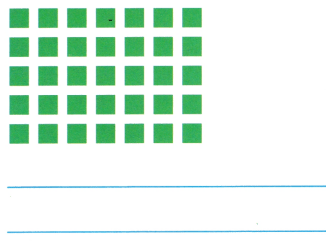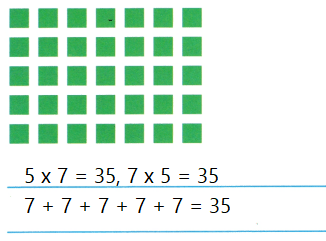Explanation:
The equations are written in three possible ways

Question 4.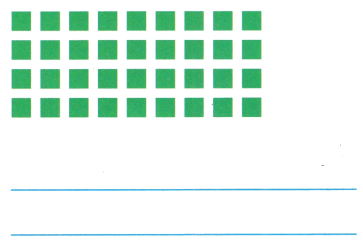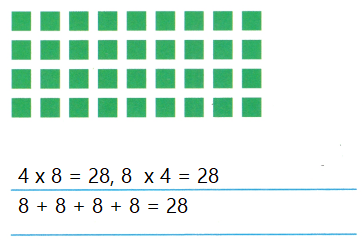Explanation:
The equations are written in three possible ways

Find the product. TEK 3.4.E, 3.4.K.

Question 5.
3 × 1 = ___
Explanation:
The product of two numbers
3 x 1 = 3

Question 6.
5 × 6 = ___
Explanation:
The product of two numbers
5 x 6 = 30

Question 7.
4 × 7 = ____
Explanation:
The product of two numbers
4 x 7 = 28

Question 8.
2 × 10 = ___
Explanation:
The product of two numbers
2 x 10 = 20

Question 9.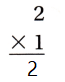Explanation:
The multiplication is done in vertical form
the product of two numbers
2 x 1 = 2

Question 10.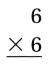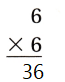Explanation:
The multiplication is done in vertical form
the product of two numbers

Question 11.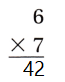Explanation:
The multiplication is done in vertical form
the product of two numbers
6 x7 = 42

Question 12.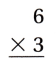Explanation:
The multiplication is done in vertical form
the product of two numbers
6 x 3 = 18

Question 13.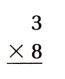Explanation:
The multiplication is done in vertical form
the product of two numbers
3 x 8 =24

Texas Test Prep

Fill in the bubble for the correct answer choice.
You may use models to solve.

Question 14.
Lori saw 6 lightning bugs. They each had 6 legs. How many legs did the lightning bugs have in all? TEKS 3.4.E, 3.4.K
(A) 36
(B) 6
(C) 12
(D) 24
Explanation:
Lori saw 6 lightning bugs.
36 legs did the lightning bugs have in all, Since 6 x 6 =36.

Question 15.
Zach gave his dog 1 dog biscuit each day, for 5 days. Then he gave his dog 2 dog biscuits a day for the next
5 days. How many biscuits did Zach give to his dog? TEKS 3.4.E, 3.4.K
(A) 5
(B) 30
(C) 15
(D) 7
Explanation:
Zach gave his dog 1 dog biscuit each day, for 5 days. So first 5days he given 5 biscuits, Since 5 x 1= 5.
he gave his dog 2 dog biscuits a day for the next 5 days. So next 5 days he given 10 biscuits, Since 5 x 2= 10.
So Total is 10 + 5 = 15.

Question 16.
Annette bought 4 boxes of pencils. If there are 8 pencils in each box, how many pencils did she buy? TEKS 3.4.E, 3.4.K
(A) 32
(B) 12
(C) 16
(D) 24
Explanation:
Annette bought 4 boxes of pencils. If there are 8 pencils in each box, 32 pencils did she buy. Since 4 x 8 =32.

Question 17.
Shelly can paint 4 pictures in a day. How many pictures can she paint in 7 days? TEKS 3.4.E, 3.4.K
Record your answer and fill in the bubbles on the grid. Be sure to use the correct place value.